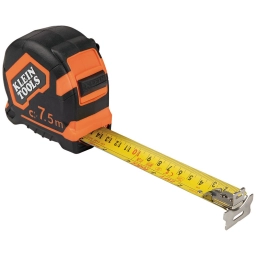# A 28-inch

A 28-inch ribbon is cut into 8 pieces that are all the same length. What is the length of each piece?

b =  7/2 = 3 12 in

### Step-by-step explanation:Did you find an error or inaccuracy? Feel free to write us. Thank you!

Tips for related online calculators
Need help calculating sum, simplifying, or multiplying fractions? Try our fraction calculator.
Do you want to convert length units?